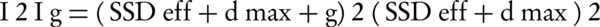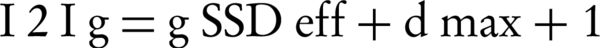# Determining the Effective Source-Surface Distance for Therapeutic Electron Beams

authors:Mohammad Javad Tahmasebi Birgani 1 , 2 ,Mansour Zabihzadeh 1 , 2 , 3 , * ,Sholeh Arvandi 2 ,Elahe Gharibreza 1

Department of Medical Physics, School of Medicine, Ahvaz Jundishapur University of Medical Sciences, Ahvaz, IR Iran
Department of Clinical Oncology, Golestan Hospital, Ahvaz Jundishapur University of Medical Sciences, Ahvaz, IR Iran
Student Research Committee, Ahvaz Jundishapur University of Medical Sciences, Ahvaz, IR lran

how to cite: Tahmasebi Birgani M J, Zabihzadeh M, Arvandi S, Gharibreza E. Determining the Effective Source-Surface Distance for Therapeutic Electron Beams. Jentashapir J Cell Mol Biol. 2016;7(3):e30975. doi: 10.17795/jjhr-30975.

### Abstract

#### Background:

The source point of the irradiated electron beam must be considered to estimate the output factor and dose distribution during electron therapy.

#### Objectives:

The aim of this study was to determine the effective source-surface distance (SSDeff) of an electron linear accelerator (Linac), and its dependence on energy and depth.

#### Materials and Methods:

A Varian Linac 2100CD with electron energies of 4, 6, 9, 12, and 15 MeV, electron applicator size of 20 × 20 cm2, nominal SSDs of 97 to 113 cm, and air gaps of 2 to 18 cm were studied. Using a Farmer (0.13 cc) ionizing chamber, the percentage depth doses were measured in the water phantom (50 cm3) and then the SSDeff was calculated by applying the inverse square law.

#### Results:

For the 100% PDD, the SSDeff values were calculated as 79, 91, 92, 93, and 92 cm for 4, 6, 9, 12, and 15 MeV, respectively. At a depth with a certain PDD, increasing energy also increases SSDeff, and a similar increase is observed at a distinctive energy by increasing the PDD.

#### Conclusions:

Using the maximum dose depth from PDD curves and the inverse square law, the required SSDeff to calculate the dose distribution of the electron beam can be calculated.

### 1. Background

Megavoltage electron beams are used for radiation treatment of shallow and superficial tumors. The source-surface distance (SSD) is one of the required parameters to calculate the output factor and dose distribution for these electron beams, and its assessment requires knowing a point as the electron source. Unlike photon beams, electron beams do not originate from a specified physical source (such as a target in photon beam radiotherapy) of the accelerator’s head. The produced electron beam passing through different components of the Linac head becomes divergent, and this creates the impression that it is generated from a single point. By back projection of the movement path of electrons along the patient surface, one can define the virtual electron source (1).

Several ways have been suggested to determine the virtual electron source. By imaging the copper grids or plates containing circular holes at various distances from the collimator and by back projection of the images of grid blades or cavity walls, their point of intersection can be regarded as a virtual source (2). The use of a virtual SSD for estimating the output factor in different SSDs can only be acceptable for the large field sizes, as for small field sizes this method gives the wrong estimated values (3). The concept of effective SSD (SSDeff) was originally proposed by Khan et al. (1978). It makes the use of the SSD factor acceptable for calculating the output factor for different gaps between the therapeutic surface and the end of the electron applicator, by measuring the depth of the maximum dose (4).

By applying corrections resulting from the SSDeff method for electron beams, Johnson et al. (1994) have shown that hot and cool spots are acceptable in terms of clinical applications (3). Xu et al. (2005) assessed the effect of different scattering conditions around different positions of the dosimeter (in the air, on the surface of the water, and the maximum depth in solid water) on the SSDeff (5). Al Asmary et al. (2010) reported the dependency of the SSDeff on the field size and electron beam energy. The estimated SSDeff for the standard applicators can be used to calculate the designed shield for electron beams (6).

Usually, incorrect estimation of output factors for SSDs different than SSD = 100 cm is one of the main sources of error when calculating dose distribution during electron therapy in radiotherapy applications. An individual tabulation of SSDseff as function of energy, depth, and field size is necessary to calculate dose distribution during clinical situations.

### 2. Objectives

The aim of this study is to determine the SSDseff of electron beams from a Varian 2100 CD Linac (established in the radiotherapy department of Golestan Hospital, Ahvaz, Iran) as a simple analytical equation, and to estimate its dependency on beam energy and water phantom depth.

### 3. Materials and Methods

A Varian Linac 2100CD with energies of 4, 6, 9, 12, and 15 MeV and an electron applicator with a size of 20 × 20 cm2 were used to dose measurements. Nominal SSDs of 97, 100, 105, 110, and 113 cm with air gaps of 2, 5, 10, 15, and 18 cm between the end of the applicator and the water phantom surface were applied. All dose measurements were carried out using Farmer ionizing chambers with a sensitive volume of 0.13 cc in the water phantom (50 cm3), a PTW-Blue Phantom with Perspex walls. A Scanditronix Wellhofer dosimetry system and Omnipro software (version 6.4) were used for dose readings. Each measurement was repeated at least three times to maintain repeatability and to ensure the stability of the output of the system.

Unlike what was supposed by Khan et al. (1978), a zero air gap (g = 0) is not possible on the Varian Linac 2100CD accompanied by an electron applicator due to structural limitations. Doses are measured in a phantom at the depth of maximum dose (dm), with the phantom first at the standard SSD (with 2 cm air gap, g = 2 cm) and then at various distances up to 18 cm from the applicator end. Suppose I2 = a dose with g = 2 cm and Ig = a dose with gap g between the standard SSD point and the phantom surface. Then, using the inverse square law:

Equation 1.(2)

By plotting √(I2/Ig) as a function of gap g, a straight line is obtained with a slope of:(3)

Finally, the effective SSD is given by:(4)

In addition to determining the SSDeff at a depth of maximum dose, the SSDseff values were drawn for the different depths extracted from the PDD curve of the Omnipro software, and their changes for the various energies and the depths were evaluated. The maximum error of average dose measurements in any depth after at least three repetitions was less than 5%.

### 4. Results

Using the inverse square law and by applying the various air gaps, the amount of √(I2/Ig) for energies of 4, 6, 9, 12, and 15 MeV for the 20 × 20 cm2 electron applicator were measured. As shown in Figure 1, √I2/Ig increases almost linearly with increase of the air gap’s height, except for the 50% PDD of 4 MeV. These fluctuations are expected due to the high dose gradient of low-energy beams at shallow depths with lower %PDDs. The slopes of these lines were extracted to calculate SSDseff (Table 1).

Table 1. Values of SSDeff in Different Depths of the PDDs of 50%, 70%, 80%, and 100% for Energies of 4, 6, 9, 12, and 15 MeV
Depth, SSDeffEnergy, MeV
4691215
100% PDD7991929392
50% PDD7079828382
70% PDD7385868887
80% PDD7685888987

### 5. Discussion

Electron beams, unlike photon beams, do not emanate from a physical source of a Linac’s head. Calculation of output factors and curves of the dose distributions resulting from the accelerator in radiotherapy applications requires determining the source of electron beam. Table 1 shows the dependency of SSDeff with measurement depth in phantom, and the energy of the electron beam. At a depth with a certain PDD, by increasing the energy the SSDeff is increased, and likewise increases by increasing PDD at related to a particular depth a distinctive energy. These results are consistent with the results reported by others (2, 7).

Ostwald et al. (1996) reported that effective source-surface distances (ESSD) varied with depth and energy of the electron beam. The surface ESSD varied from 68 cm for 4 MeV to 82 cm for 16 MeV, but increased with depth to a maximum value, which was found at approximately half the practical range (Rp) (7). The use of less PDD and less energy causes lower SSDeff values. Changes in SSDeff for energies higher than 6 MeV are not noticeable. This indicates that for the exact dose calculation using electron beams of lower energies like 4 MeV, the related SSDeff has a lot of differences from that in the higher energies. As reported by Rajasekar et al. (2002), the rapid reduction of dose due to the applicator-scattered component with an extended SSD was significant for the lower energies (8).

The average difference of SSDeff (for all the energies using PDD of 50%, 70%, and 80%) in comparison with the PDD of 100% was about 12%, 6%, and 5%, respectively which is not acceptable for calculating SSDeff. These differences are mainly caused by decreased output at smaller depths. A difference of 31 cm between the surface ESSD and the maximum ESSD (for the SSDs of 100 - 130 cm and for the 20 MeV beam in the 6 × 6 cm2 field) was reported by Ostwald et al. (1996) (7). Hence, in agreement with their result, the SSDeff calculated at the maximum dose depth (Dmax) may be used with reasonable accuracy for calculating the dose in the therapeutic range.

The amount of SSDeff is dependent on energy and the depth of the selected PDD. At a given energy by changing the depth of the selected PDD, the SSDeff shows considerable change. By using the PDD in the depth of maximum dose, the required SSDeff can be calculated by applying the inverse square law.

### References

• 1.

Variability in the position of the virtual source for small clinical electron beams. Medical Physics. : 1926

• 2.

O'Shea T, Foley MJ, Rajasekar D, Downes PA, Van der Putten W, Moore M, et al. Electron beam therapy at extended source-to-surface distance: a Monte Carlo investigation. J Appl Clin Med Phys. 2008; 9 (4) : 2811 [PubMed]

• 3.

Johnson JM, Khan FM. Dosimetric effects of abutting extended source to surface distance electron fields with photon fields in the treatment of head and neck cancers. Int J Rad Oncol Biol Phys. 1994; 28 (3) : 741 -7

• 4.

Khan FM, Sewchand W, Levitt SH. Effect of air space and depth dose in electron beam therapy. Radiology. 1978; 126 (1) : 249 -51 [DOI][PubMed]

• 5.

Xu D, Xiao Y, Nawaz O, Galvin J. SU‐FF‐T‐173: A Comparison of Techniques for Effective SSD Measurements and Evaluation of Data for Accelerators From Different Manufactures. Med Phys. 2005; 32 (6) : 1989

• 6.

Al Asmary M, Ravikumar M. Position of effective electron source for shielded electron beams from a therapeutic linear accelerator. PJMPE. 2010; 16 (1)[DOI]

• 7.

Ostwald PM, Kron T. Variation in calculated effective source-surface distances with depth. Phys Med Biol. 1996; 41 (10) : 2067 -78 [PubMed]

• 8.

Rajasekar D, Datta NR, Das KJ, Ayyagari S. Electron beam therapy at extended SSDs: an analysis of output correction factors for a Mitsubishi linear accelerator. Phys Med Biol. 2002; 47 (18) : 3301 -11 [PubMed]

Copyright © 2016, Ahvaz Jundishapur University of Medical Sciences. This is an open-access article distributed under the terms of the Creative Commons Attribution-NonCommercial 4.0 International License (http://creativecommons.org/licenses/by-nc/4.0/) which permits copy and redistribute the material just in noncommercial usages, provided the original work is properly cited.